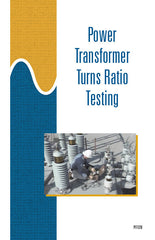#### Get the Study Guide for this videoFollow the video session-by-session with this handy book. Color illustrations throughout. \$15

Instructor Guide  available

# Power Transformer Turns Ratio Testing - Instant Video

##### Summary

This 32-minute DVD explains what a transformer turns ratio test is, how the test is performed, and what the results of the test mean. A substation power transformer is designed to step up voltage for transmission over long distances or to step down voltage for distribution to homes and businesses. The change in voltage depends on the ratio of the transformer’s primary winding to its secondary winding. Some transformer winding problems can be detected while they are still minor by using a test known as a transformer turns ratio test.

##### Training Sessions

The Purpose of Transformer Turns Ratio Testing – 4 minutes
Explains what a transformer turns ratio is. Describes how the turns ratio of a transformer can be changed. Describes the purpose of transformer turns ratio testing.

Test Equipment – 9 minutes
Explains the principle of turns ratio testing. Identifies the components of a typical turns ratio test set and describes the function of each component.

Test Connections – 6 minutes
Identifies the connections for testing the turns ratio of a delta-wye power transformer.

Test Procedures – 6 minutes
Describes safety precautions commonly observed during turns ratio testing. Describes the procedure for performing a turns ratio test on a power transformer.

Evaluating Test Results – 7 minutes
Describes what the following turns ratio test results indicate about the windings of the transformer under test: High exciting current and low generated voltage, normal exciting current, normal exciting voltage but no null meter needle deflection. Demonstrates how to calculate the expected turns ratio for a transformer. Identifies a typical acceptable turns ratio error.

Item Code: PTT-IV